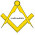# How to Find Circumference and Area of a Circle

Before, we talk about area and circumference of a circle. Let's find out what is diameter and π? The diameter of a circle is any straight line segment that passes through the center of the circle. The number π is a mathematical constant, the ratio of a circle's circumference to its diameter. Commonly approximated as 3.14159. It has been represented by the Greek letter "π" since the mid-18th century, though it is also sometimes spelled out as "pi". Being an irrational number, π cannot be expressed exactly as a fraction (equivalently, its decimal representation never ends and never settles into a permanent repeating pattern). Still, fractions such as 22/7 and other rational numbers are commonly used to approximate π.

### Calculate the Circumference of a Circle

We already know that the ratio of the circumference of a circle to its diameter is π, written
π = C/d
From the equation above, we obtain  the following equation
C = πd
Since d = 2r, then
C = 2πr
So, for each circle the formula of circumference in the following is valid
C = πd or C =2πr
where:
C = circumference of a circle
π = 3.14 or 22/7
d = diameter of the circle
r = radius of the circle

Example 1
The diameter of a coin 2.8 cm. Calculate its circumference!
Since it is given that the length of the diameter is multiple of 7, then to simplify the calculation use π = 22/7
C = πd
C = 22/7 x 2.8
C = 8.8 cm
So, the circumference of the coin is 8.8 cm

Example 2
The radius of a car tire is 25 cm. Calculate the circumference of the car tire!
Since it is given that the length of the radius is not multiple of 7, then to simplify the calculation use π = 3.14
C = 2πr
C = 2 x 3.14 x 25
C = 157 cm
So, the circumference of the car tire is 157 cm

### Calculating the Area of a Circle

To find the formula of a circle area, look at the following figure
Consider the rectangle obtained from the circle. Lenght of the rectangle is half of the circle circumference (πr) and the width is equal to the radius of the circle (r). The area of the circle is also equal to the area of the rectangle. From these, we obtain the following equation
The area of the circle = The area of the rectangle
A = length x width
A = πr x r
A = πr

If the area expressed in its diameter, then:
A = πr
A = π(1/2 d)
A = 1/4 πd

From the explanation above we can conclude that for each circle, the area of a circle in the following is valid
A = πr or A = 1/4 πd
where
A = the area of the circle
π = 3.14 or 22/7
r = radius of the circle
d = diameter of the circle

Example 3
Calculate the area of a circle of 88 cm in circumference and π = 22/7
From the circumference we calculate the radius
C = 2πr
C = 88 cm
2πr = 88
2 x 22/7 x r = 88
r = 88 x 7/44
r = 14 cm

the, calculate the area
A = πr
A = 22/7 x 14 x 14
A = 616 cm
So, the area of the circle is 616 cm

Example 4
Mr. Obama will make a flower park in the form of a circle on a rectangular land each side of which is 20 m long. If the park is made as large as possible then find the area of the park.
To make the park as large as possible, diameter of the park = 20 m
A = 1/4  πd
A = 1/4 3.14 x 20
A = 314 m
So, area of the park is 314 m

Berlangganan update artikel terbaru via email:

### 1 Response to "How to Find Circumference and Area of a Circle "

1.